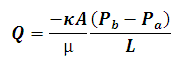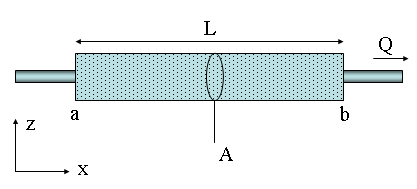# Darcy's Law Calculator

This Calctown Calculator calculates the instantaneous discharge rate through a porous medium at constant elevation using Darcy's law.

m2
m2
Pa.s
Pa
Pa
m

#### Result

m3/swhere

Q = discharge rate

κ = intrinsic permeability of medium

μ = viscosity

P = pressure

L = path length

A = cross-sectional area